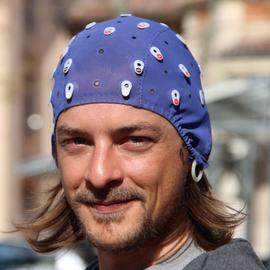# Lecture on Linear Mixed Models

Introduction

This is a series of lectures I gave to doctoral students in psychology and neuroscience at Aix-Marseille Université in April 2021. The course aimed at giving enough information and examples about mixed models to allow students to think about how they can apply such models to their data.

All the course is intended to be “hands-on” and therefore the R code is provided with reproducible examples (mainly simulations). The code is meant to be accessible to beginners so it remains simple and redundant (and… I’m a python user so I don’t get all the tidyverse things for now).

All the content of the slides can be recreated as an interactive jupyter notebook where you can easily live code inside the presentation thanks to the RISE python package. If you’re interested in replicating this lecture take a look at the root repository (https://github.com/GWeindel/lecture_mixed_models_AMU_2021) with all the instructions.

Content of the course :

Module 1 The first module is dedicated to linear models both as a reminder and to extend students knowledge on what can be done using linear models (prediction, factor recoding, etc.)

The presentation can be found here (best to open in a separate tab and to navigate across slides with the space bar)

Module 2 The second module introduces the core aspect of linear mixed models in a frequentist context and using the ‘‘’lme4’’’ R package. We start by illustrating the concept of maximum likelihood, hierarchies in the data and how to account for these hierarchies.

The presentation can be found here

Module 3 The last module introduces Bayesian estimation and generalized linear mixed models. The aim is to give students clues to understand these models fitted in a Bayesian context rather than being exhaustive, I conclude with a series of resources for those who want to go further in the learning of regression models and Bayesian estimation.

The presentation can be found here##### Gabriel Weindel
###### Post-doctoral researcher

My research interests is mental chronometry, the time taken by information processing units in our brain. To study these times I rely on cognitive models of behavior that I combine with neural data such as electro-encephalography.